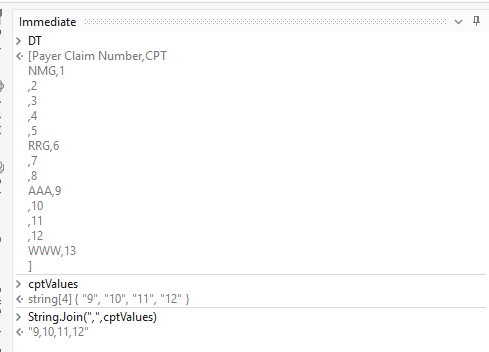Hi all

I need to get CPT codes (f2,f3,f4,f5,f6,f7) and Amount(K2,k3,k4.k5.k6.k7)belong to per claim number(B2) and will save into a string variable with Table format for CPT codes and amount . It may vary per claim numbers

Can anyone please let me know how to do it?

Hi @divya.17290 ,

Could you try with the Steps below :

1. Read the Data from Excel using `Read Range` Activity, Get the Output as Datatable.

2. Next, We use `Look Up Datatable` Activity to get the Index of the row and value of CPT for the matching Claim Number.

3. Next, We can use Skip and Take Linq methods to get the rows we need and then select the CPT Values with the help of below Linq :

``````cptValues = {cptValue}.Concat(DT.AsEnumerable.Skip(rowIndex+1).TakeWhile(Function(x)String.IsNullOrWhiteSpace(x("Payer Claim Number").ToString)).Select(Function(x)x("CPT").ToString).ToArray).ToArray
``````

Here, `cptValue` and `rowIndex` are the Output values from `Look Up Datatable` Activity. `DT` is the input datatable and `cptValues` is a variable of type Array of String.

1. We can then Convert or Combine the array values in the form of a String using `String.Join` :
``````String.Join(",",cptValues)
``````

Output :Let us know if you are facing any issues.

4 Likes

Thank you, i can get the CPT codes.

1 Like

Hi
I m facing one issues while reading the rows in lookup table " Lookup Data Table: StringConverter cannot convert from System.Double".
I have the value in PayerClaimNumber = “1.234E+12” in Excel , Please let me know how do i read this value.

@divya.17290 ,
Could you try changing the variable type of `Lookup Datatable` Activity’s `cellValue` property to `UiPath.Core.GenericValue` and check if it works ?

This topic was automatically closed 3 days after the last reply. New replies are no longer allowed.